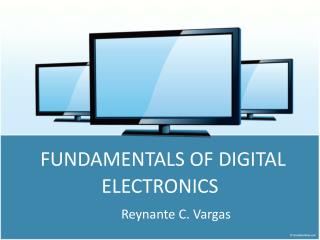# Fundamentals of Digital Electronics - PowerPoint PPT PresentationDownload PresentationFundamentals of Digital Electronics

Fundamentals of Digital Electronics
Download Presentation## Fundamentals of Digital Electronics

- - - - - - - - - - - - - - - - - - - - - - - - - - - E N D - - - - - - - - - - - - - - - - - - - - - - - - - - -
##### Presentation Transcript

1. FUNDAMENTALS OF DIGITAL ELECTRONICS Reynante C. Vargas

2. LOGIC GATES A gate is a circuit which can have any number of inputs but only one output. These gates are the fundamental of flip flops which is used basically in computers. Logic gates process signals which represent true or false. Normally the positive supply voltage +Vs represents true and 0V represents false.

3. KINDS OF LOGIC GATES • AND GATE • NOT GATE • OR GATE • NAND GATE / NOT AND GATE • NOR GATE / NOT OR GATE

4. AND GATE • This kind of gate requires 1 as input to all its inputs in order to produce an output of 1. Where: A and B are inputs Q is output Symbol of AND Gate

5. AND GATE TRUTH TABLE • The output Q is true if input A AND input B are both true: Q = A AND BAn AND gate can have two or more inputs, its output is true if all inputs are true.

6. A Q input output NOT GATE • This is a very special type of gate which is also called inverter. • Whatever may be the input the output will be just the opposite. Where: A is input Q is output Symbol of NOT Gate

7. A Q input output NOT TRUTH TABLE The output Q is true when the input A is NOT true, the output is the inverse of the input: Q = NOT AA NOT gate can only have one input.

8. A Q inputs output B OR GATE • This gate functions as the addition of the two Binary Inputs. • An OR gate can have two or more inputs, its output is true if at least one input is true. Where: A and B are inputs Q is output Symbol of OR Gate

9. A Q inputs output B OR GATE TRUTH TABLE • The output Q is true if input A OR input B is true (or both of them are true): Q = A OR BAn OR gate can have two or more inputs, its output is true if at least one input is true.

10. A Q inputs output B NAND GATE • NAND gate (NAND = Not AND) • The NAND GATE functions by multiplying the binary inputs but the output is the opposite of the result. • This is an AND gate with the output inverted, as shown by the 'o' on the output.  Where: A and B are inputs Q is output Symbol of NAND Gate

11. A Q inputs output B NAND GATE TRUTH TABLE • The output is true if input A AND input B are NOT both true: Q = NOT (A AND B)A NAND gate can have two or more inputs, its output is true if NOT all inputs are true.

12. A Q inputs output B NOR GATE This gate is just the opposite of OR Gate. The outputs of this gate are just the opposites of the outputs OR Gate. This is an OR gate with the output inverted, as shown by the 'o' on the output.  Where: A and B are inputs Q is output Symbol of NOR Gate

13. A Q inputs output B NOR TRUTH TABLE • The output Q is true if NOT inputs A OR B are true: Q = NOT (A OR B)A NOR gate can have two or more inputs, its output is true if no inputs are true.

14. LOGIC GATES SYMBOL

15. EXERCISE #1: Complete the truth table of the logic gate below

16. EXERCISE #2: Make a truth table of the following logic gate.